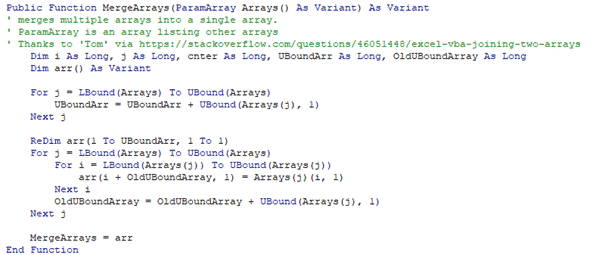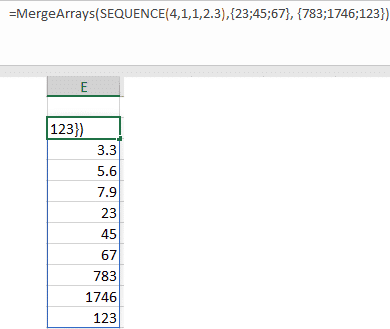# Excel merge arrays into a single array with VBA

One annoying omission from Excel and VBA is a simple way to combine various arrays into a single array.

It’s something we’ve needed to make NetworkDays() and NetworkDays_Intl() work properly in the real world with a combination of date arrays needed.

There are many merge array functions around the forums.  We’ve chosen this one from ‘Tom’  which merges two or more arrays at once.  All the arrays need to have the same dimension i.e  1 column, 2 column etc.In Excel MergeArrays() UDF function works like this:

`=MergeArrays(SEQUENCE(4,1,1,2.3),{23;45;67}, {783;1746;123})`with Range2Array() you can combine ranges with arrays into a single array.

`=MergeArrays({23;45;67}, Range2Array(B4:B14))`

All the array elements are combined in the order of the parameters.  There are Sort() SortBy() and Unique() functions that can handle management of the merged array.

## Direct References

A problem we found with all the suggested merge array UDF’s is that direct references to arrays returned a #Value error in Excel 365 for Windows.We could not figure out why. All the parameters are the same type.  Maybe an Excel bug but more likely some subtlety in VBA.  If you can shed light on this mystery, please let us know.

The workaround is to pass in the array wrapped around a ‘harmless’ array function like Sort().  That workaround is OK for our purposes with NetworkDays().

`=MergeArrays(SORT(A2#),SORT(B2#))`Excel convert Range to Array in VBA

Excel now has Dynamic Arrays – Windows, Mac and more …

Excel Array formulas for everyone

```Public Function MergeArrays(ParamArray Arrays() As Variant) As Variant

' merges multiple arrays into a single array.

' ParamArray is an array listing other arrays

' Thanks to 'Tom' via https://stackoverflow.com/questions/46051448/excel-vba-joining-two-arrays

Dim i As Long, j As Long, cnter As Long, UBoundArr As Long, OldUBoundArray As Long

Dim arr() As Variant

For j = LBound(Arrays) To UBound(Arrays)

UBoundArr = UBoundArr + UBound(Arrays(j), 1)

Next j

ReDim arr(1 To UBoundArr, 1 To 1)

For j = LBound(Arrays) To UBound(Arrays)

For i = LBound(Arrays(j)) To UBound(Arrays(j))

arr(i + OldUBoundArray, 1) = Arrays(j)(i, 1)

Next i

OldUBoundArray = OldUBoundArray + UBound(Arrays(j), 1)

Next j

MergeArrays = arr

End Function```
Don't miss out!
Join Office for Mere Mortals

Office for Mere Mortals is where thousands pick up useful tips and tricks for Word, Excel, PowerPoint and Outlook.